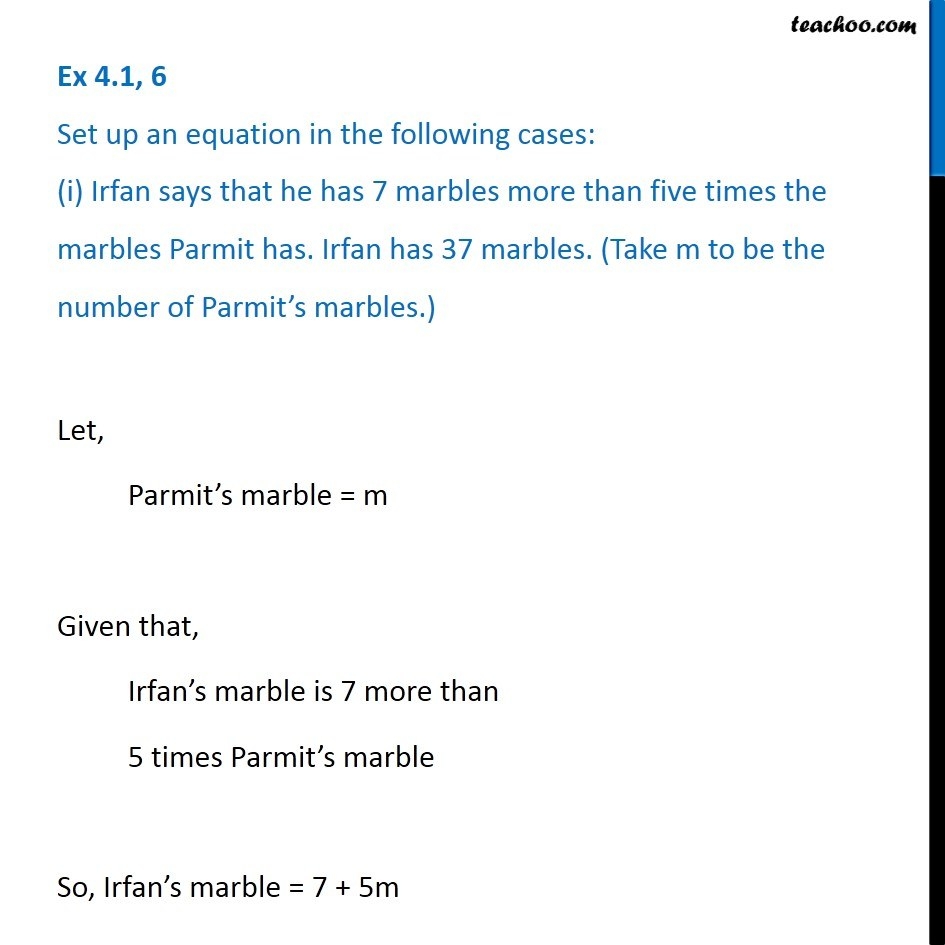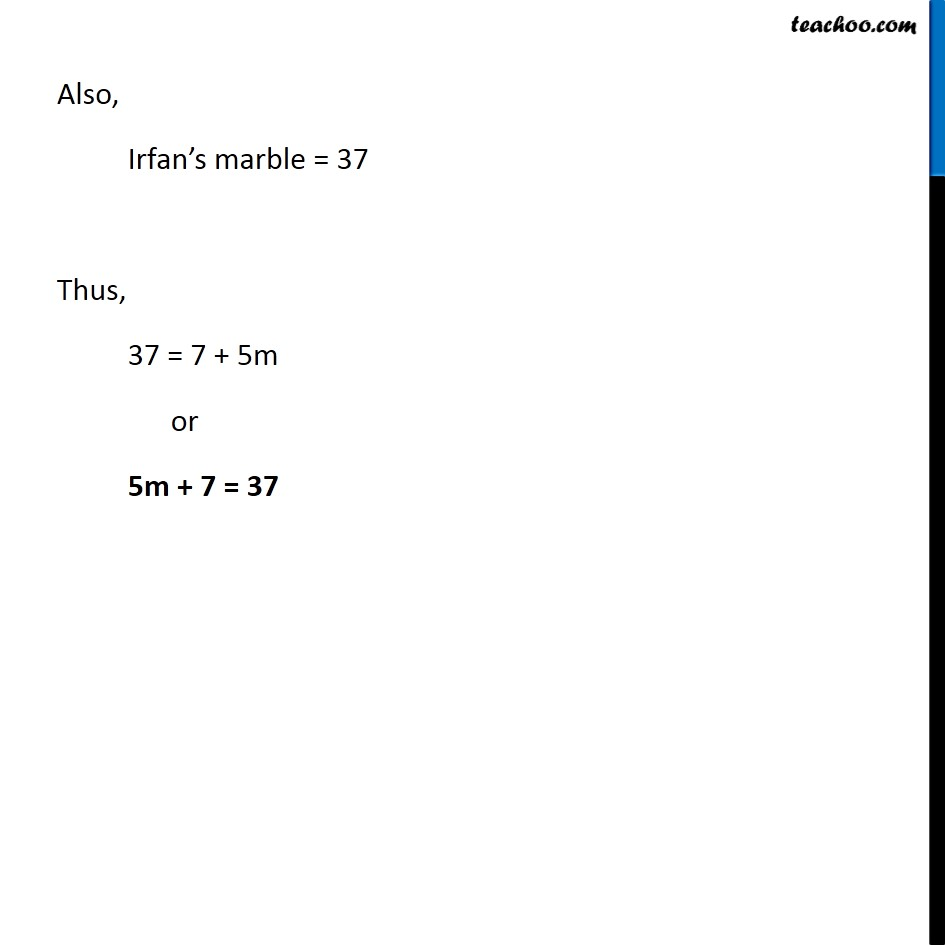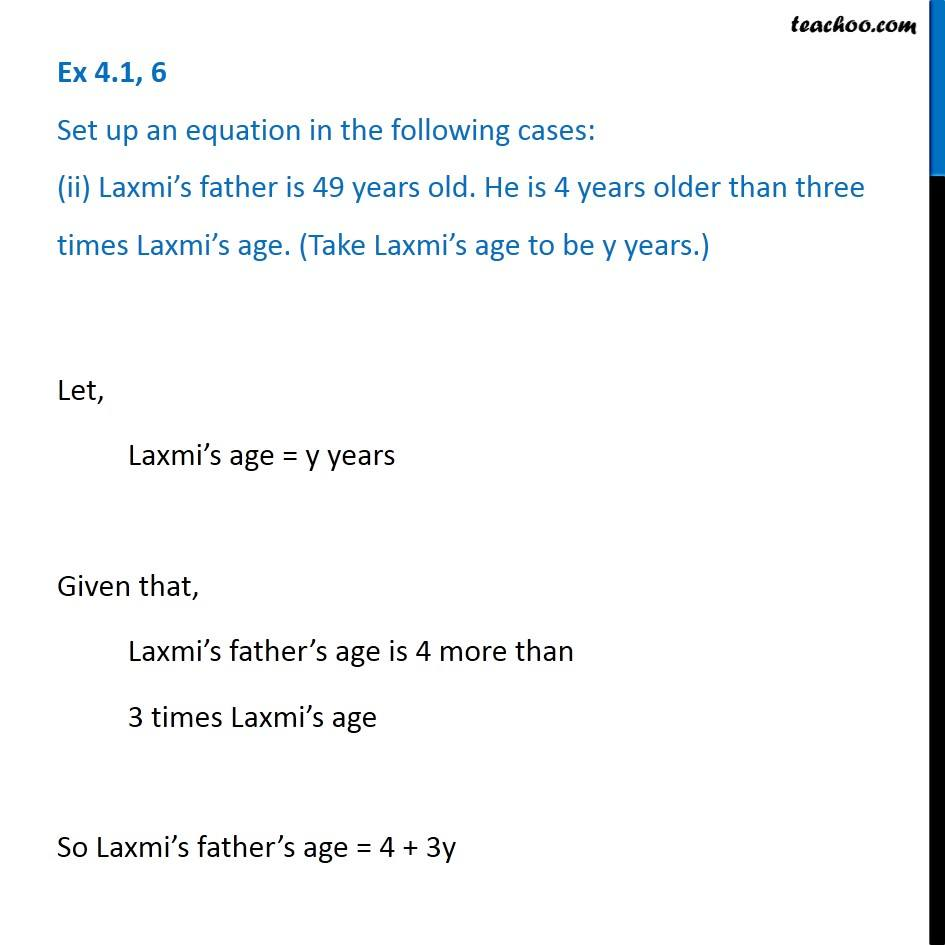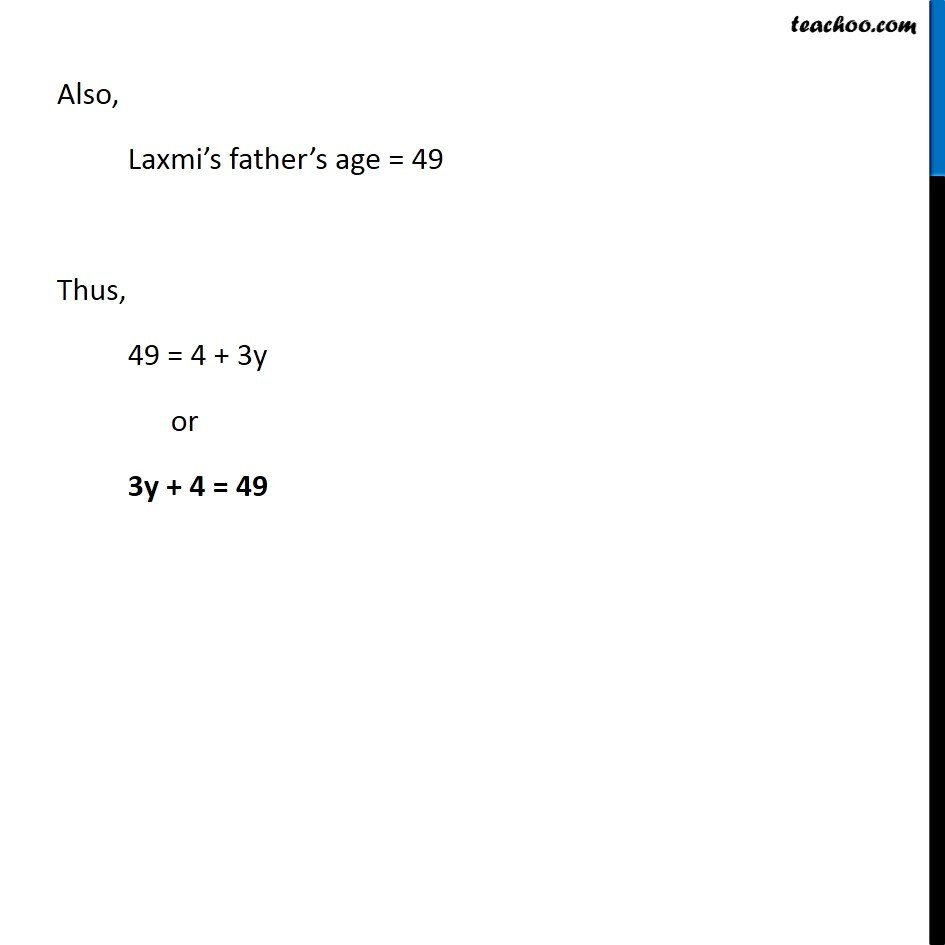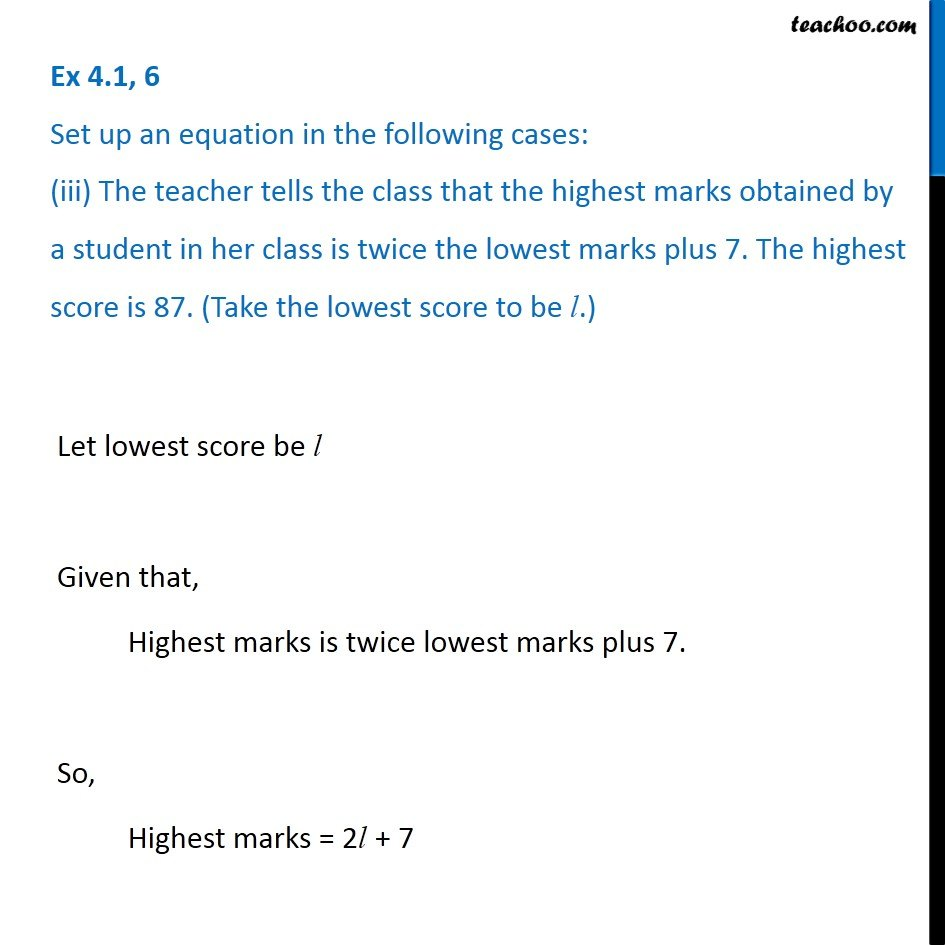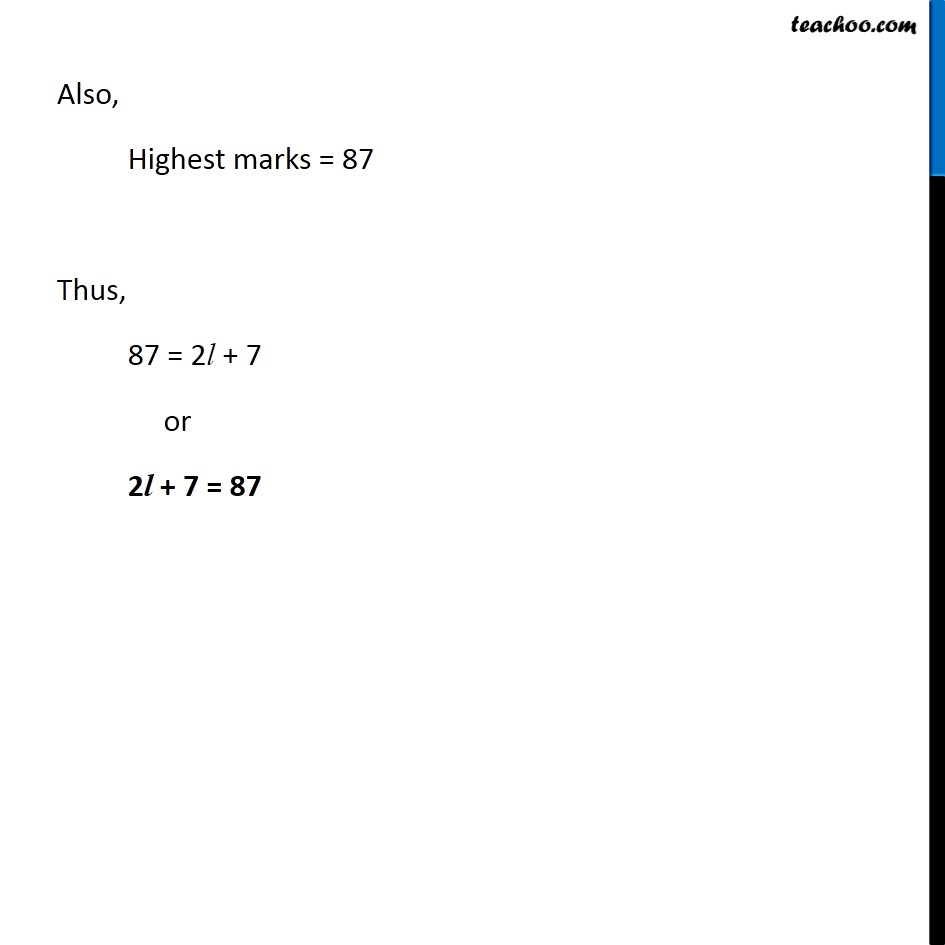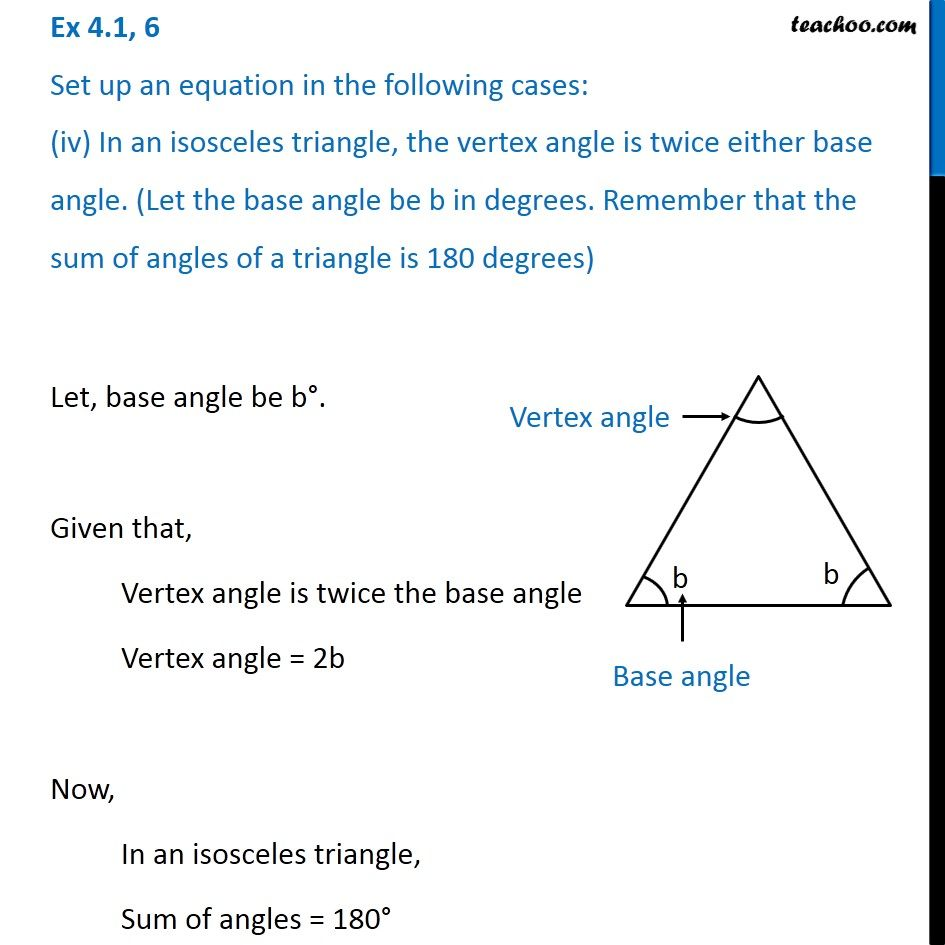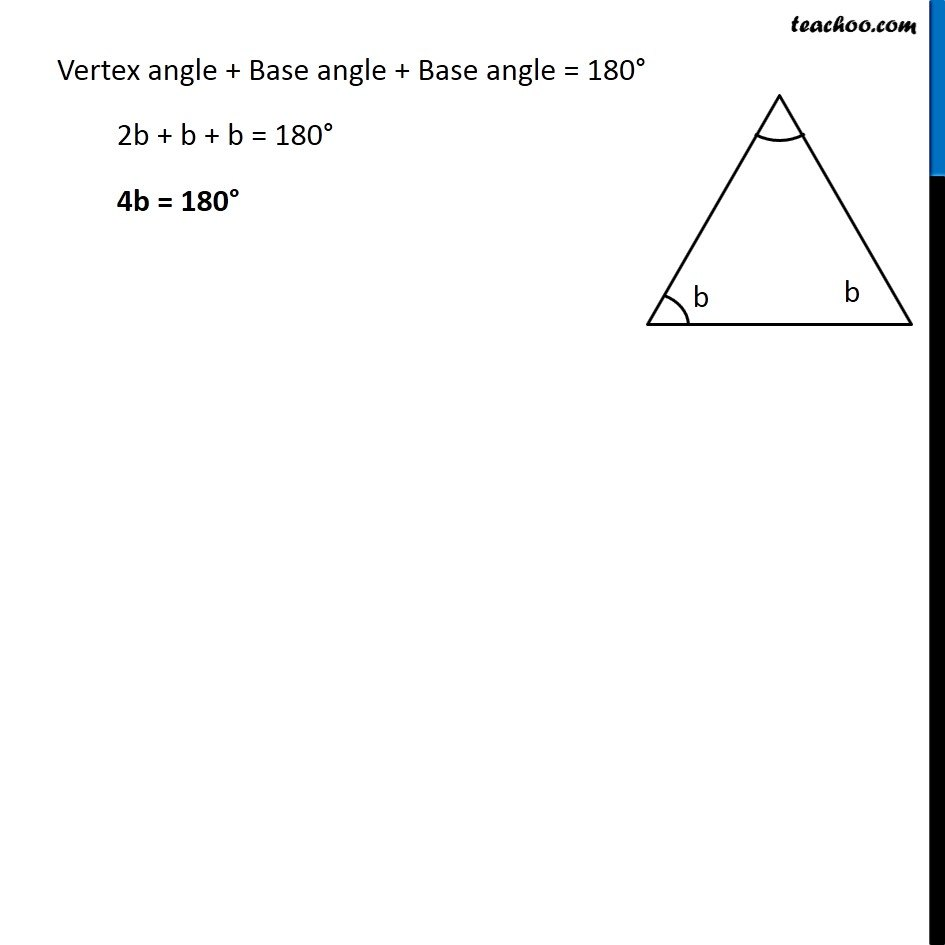Subscribe to our Youtube Channel - https://you.tube/teachoo

1. Chapter 4 Class 7 Simple Equations
2. Serial order wise
3. Ex 4.1

Transcript

Ex 4.1, 6 Set up an equation in the following cases: (i) Irfan says that he has 7 marbles more than five times the marbles Parmit has. Irfan has 37 marbles. (Take m to be the number of Parmit s marbles.)Let, Parmit s marble = m Given that, Irfan s marble is 7 more than 5 times Parmit s marble So, Irfan s marble = 7 + 5m Also, Irfan s marble = 37 Thus, 37 = 7 + 5m or 5m + 7 = 37 Also, Irfan s marble = 37 Thus, 37 = 7 + 5m or 5m + 7 = 37 Ex 4.1, 6 Set up an equation in the following cases: (ii) Laxmi s father is 49 years old. He is 4 years older than three times Laxmi s age. (Take Laxmi s age to be y years.)Let, Laxmi s age = y years Given that, Laxmi s father s age is 4 more than 3 times Laxmi s age So Laxmi s father s age = 4 + 3y Also, Laxmi s father s age = 49 Thus, 49 = 4 + 3y or 3y + 4 = 49 Ex 4.1, 6 Set up an equation in the following cases: (iii) The teacher tells the class that the highest marks obtained by a student in her class is twice the lowest marks plus 7. The highest score is 87. (Take the lowest score to be l.) Let lowest score be l Given that, Highest marks is twice lowest marks plus 7. So, Highest marks = 2l + 7 Also, Highest marks = 87 Thus, 87 = 2l + 7 or 2l + 7 = 87 Ex 4.1, 6 Set up an equation in the following cases: (iv) In an isosceles triangle, the vertex angle is twice either base angle. (Let the base angle be b in degrees. Remember that the sum of angles of a triangle is 180 degrees) Let, base angle be b . Given that, Vertex angle is twice the base angle Vertex angle = 2b Now, In an isosceles triangle, Sum of angles = 180 Vertex angle + Base angle + Base angle = 180 2b + b + b = 180 4b = 180

Ex 4.1